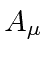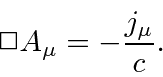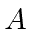## Gauge Invariance can Simplify Equations

We have already studied many aspects of gauge invariance in electromagnetism and the corresponding invariance under a phase transformation in Quantum Mechanics. One point to note is that, with our choice to treat each component ofas an independent field'', we are making a theory for the vector fieldwith a gauge symmetry, not really a theory for the field.

Recall that the gauge symmetry of Electricity and Magnetism and the phase symmetry of electron wavefunctions are really one and the same. Neither the phase of the wavefunction nor the vector potential are directly observable, but the symmetry is.

We will not go over the consequences of gauge invariance again here, but, we do want to use gauge invariance to simplify our equations.

Maxwell's equation isWe can simplify this basic equation by setting the gauge according to the Lorentz condition.The gauge transformation needed isThe Maxwell equation with the Lorentz condition now readsThere is still substantial gauge freedom possible. The second derivative ofis set by the Lorentz condition but there is still freedom in the first derivative which will modify. Gauge transformations can be made as shown below.This transformation will not disturb the Lorentz condition which simplifies our equation. We will use a further gauge condition in the next chapter to work with transverse fields.

Jim Branson 2013-04-22